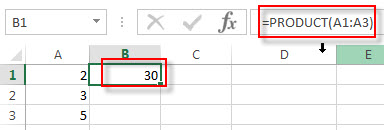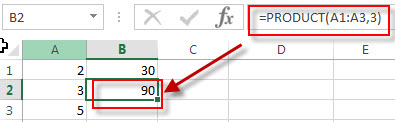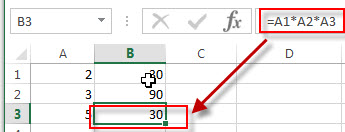# Excel PRODUCT Function

This post will guide you how to use Excel PRODUCT function with syntax and examples in Microsoft excel.

### Description

The Excel PRODUCT function multiplies the numbers and returns the product. For example, If you want to multiple the numbers in both A1 and A2 cells, and you can use the PRODUCT function to create an Excel formula =PRODUCT (A1, A2).

Of course, you can also use the multiply mathematical operator to perform a multiply operation for two numbers.

Like this: =A1*A2

The PRODUCT function is a build-in function in Microsoft Excel and it is categorized as a Math and Trigonometry Function.

The PRODUCT function is available in Excel 2016, Excel 2013, Excel 2010, Excel 2007, Excel 2003, Excel XP, Excel 2000, Excel 2011 for Mac.

### Syntax

The syntax of the PRODUCT function is as below:

`=PRODUCT (number1, [number2], ...)`

Where the PRODUCT function arguments are:

• number1 –  This is a required argument. The first number that you want to multiply.
• number2,… – This is an optional argument. The additional numbers that you want to multiply. You can enter up to 255 number arguments in the Excel 2007 and later. But it only accept up to 30 numbers in Excel 2003.

Note:

• If the number arguments cannot be treated as numeric values, the PRODUCT function will return the #VALUE! Error.

### Excel PRODUCT Function Examples

The below examples will show you how to use Excel PRODUCT Function to return the product of a supplied set of numbers.

1# to multiply the numbers in Cell A1:A3, enter the following formula in Cell B1.

`=PRODUCT(A1:A3)`2# to multiply the numbers in Cell A1:A3, and then multiplies that result by 3, enter the following formula in Cell B2.

`=PRODUCT(A1:A3,3)`3# to multiply the numbers in Cell A1:A3 by using mathematical operators instead of the PRODUCT function, enter the following formula in Cell B3.

`=A1*A2*A3`### Related Functions

• Excel SUMPRODUCT function
The Excel SUMPRODUCT function multiplies corresponding components in the given one or more arrays or ranges, and returns the sum of those products.The syntax of the SUMPRODUCT function is as below:= SUMPRODUCT (array1,[array2],…)…